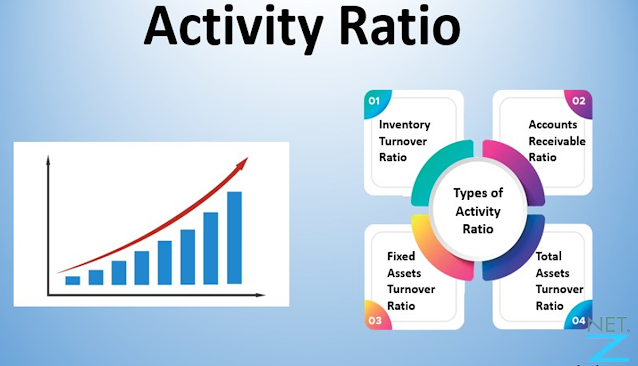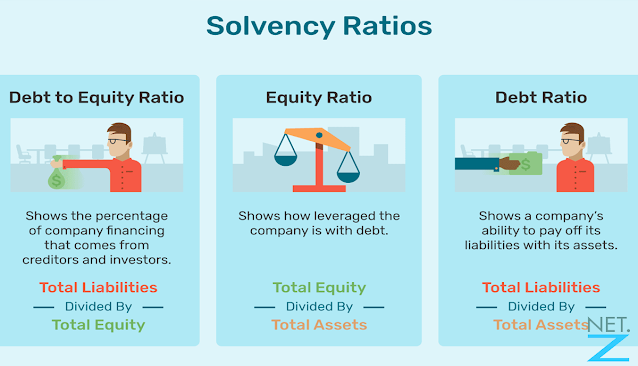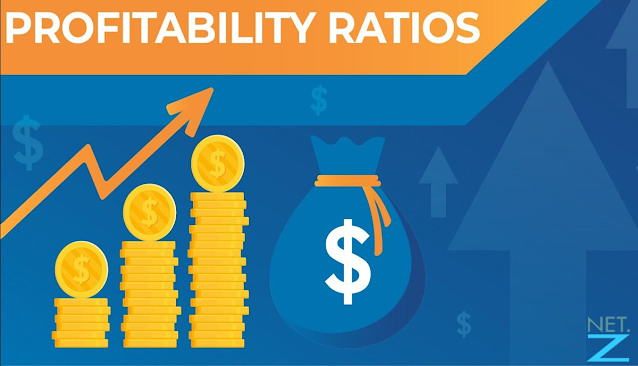# Here are some Financial Ratios that we Currently use

## Types of Financial Ratios## Ratio Analysis

Netgenz - In running a business, financial ratio analysis is important and must be done. Financial ratios are a company's financial analysis tool that is useful for assessing the performance of a company, based on a comparison of financial data contained in financial statements such as balance sheets, profit/loss reports, cash flow reports.

In general, financial ratios are used by management and investors. For investors, financial ratios are used to determine how much investment can be given. For management, financial ratios are used to determine how well the company's performance is for further evaluation. also read How surveysay to make money online.

Also Read: How surveysay to make money online

Analysis of financial ratios is not only done by hand but is carried out based on their respective types. The following are the types of financial ratios, ranging from liquidity ratios to investment ratios.

## 1. Liquidity Ratio## Liquidity ratio

Read Also: Four Basic Principles Before You Trade Crypto

The liquidity ratio is a ratio that measures the short-term liquidity ability of a company, by looking at the company's current assets against its current liabilities. That is, this ratio shows the company's ability to meet obligations or pay short-term debt.

This ratio can be analyzed using the following formula:

• Current ratio
• The formula is: (Current assets: Current liabilities) x 100%
• Fast ratio
• The formula is: [(Current assets – current inventory / current liabilities ] x 100%
• cash ratio (cash ratio)
• The formula is: Cash ratio = [(Cash + cash equivalents): current debt] x 100%

## 2. Activity Ratio## Activity ratio

Activity ratio analysis is a ratio that measures the effectiveness of a company to utilize all the resources they have so that it can be determined how high the level of activity of these assets is in certain activities.

Low assets on certain sales will result in greater excess funds embedded in these assets. If this condition occurs, the excess funds should be invested in other more productive assets.

The formula for calculating this ratio is:

• Debt Turnover = Net Sales: Average debt
• Inventory turnover = Cost of goods sold: average inventory
• Fixed asset turnover = Sales: total assets
• Total asset turnover = Sales: total assets

## 3. Solvency Ratio## Solvency Ratio

This ratio is also known as the leverage ratio, this ratio is a ratio that is used in order to assess the ability of a company to pay off debt and all its obligations, using guaranteed capital and assets (wealth in any form) owned in the long term and long term. short.

The solvency ratio is divided into two different formulas that are used to calculate the solvency ratio, including:

• Receivables to Assets Ratio (Total Debt to Asset Ratio)
• The formula is: (Total debt: total assets) x 100%
• Debt to equity ratio (Total Debt to Equity Ratio)
• The formula is: (Total debt: Capital) x 100%.

## 4. Profitability Ratio## Profitability Ratio

This type of financial ratio is used to measure and see the company's ability to generate a profit for the company. Profitability ratios are important for the survival of the company because the heart of the company will depend on the extent to which the company can benefit.

Profitability ratio analysis can be done in the following ways:

• Gross Profit Margin (Gross Profit Margin)
• The formula is: (Net profit: sales x 100%)
• Operating Profit Margin (Operating Profit Margin)
• The formula is: (Gross profit: net sales x 100%)
• Net Profit Margin (Net Profit)
• The formula is: (Net profit after tax: net sales x 100%)
• Return On Investment (ROI)
• The formula is: (Net profit after interest and taxes: Investment x 100%)
• Economic Profitability (Return On Assets)
• The formula is: (Profit before tax: total assets x 100%)

Check Other  Stock Crypto

## 5. Investment Ratio## Investment Ratio

Investment ratio is a ratio that measures the company's ability to provide returns or rewards to funders, especially investors in the capital market within a certain period of time.

This analysis aims to make potential investors know the ability of assets that will generate operating profits, and come from the company's main activities by utilizing its operating assets.